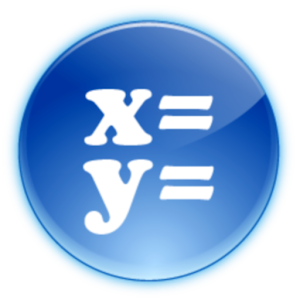# Linear System - Lumos Educational App Store1
Price -
\$

#### DESCRIPTION:

The application solves systems of equations with two or three unknowns. Each solution step by step showing what action has been taken to achieve a result. Four methods for solving systems:...

#### OVERVIEW:

Linear System is a free educational mobile app By .It helps students in grades 8 practice the following standards 8.EE.C.7.A,8.EE.C.7.B,8.EE.8.A.

This page not only allows students and teachers download Linear System but also find engaging Sample Questions, Videos, Pins, Worksheets, Books related to the following topics.

1. 8.EE.C.7.A : Give examples of linear equations in one variable with one solution, infinitely many solutions, or no solutions. Show which of these possibilities is the case by successively transforming the given equation into simpler forms, until an equivalent equation of the form x = a, a = a, or a = b results (where a and b are different numbers)..

2. 8.EE.C.7.B : Solve linear equations with rational number coefficients, including equations whose solutions require expanding expressions using the distributive property and collecting like terms..

3. 8.EE.8.A : Understand that solutions to a system of two linear equations in two variables correspond to points of intersection of their graphs, because points of intersection satisfy both equations simultaneously..

8

#### STANDARDS:

8.EE.C.7.A
8.EE.C.7.B
8.EE.8.A
8.EE.C.8.B
8.EE.C.8.C

Developer:

Software Version:

Category:

### RELATED APPSEdSearch WebSearch